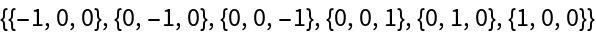Function Repository Resource:

SelectTuples

Generate tuples that satisfy a certain criterion

Contributed by: Sander Huisman
 ResourceFunction["SelectTuples"][list,n,crit] generates a list of n‐tuples of elements from list that satisfy crit. ResourceFunction["SelectTuples"][{list1,list2,…},crit] generates a list of all possible tuples whose ith element is from listi that satisfy crit. ResourceFunction["SelectTuples"][…,crit,m] picks at most the first m tuples that satisfy crit.

Details and Options

ResourceFunction["SelectTuples"] is used in cases for which generating all tuples would be too memory intensive, especially when the elements of list are large and the number of tuples that satisfy crit is small.
ResourceFunction["SelectTuples"] is an alternative to Select[Tuples[],crit], which first generates all tuples.

Examples

Basic Examples

Find out for which 2-tuple the sum is a prime:

 In:=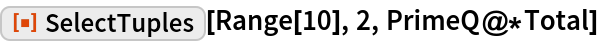Out=Only get the first five:

 In:=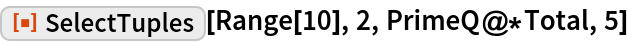Out=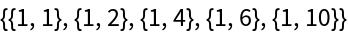Scope

Find the first 15 three-letter palindromic lists:

 In:=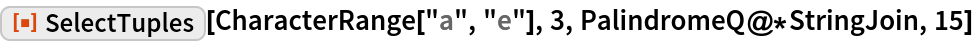Out=Applications

Find vectors for which the norm is an integer:

 In:=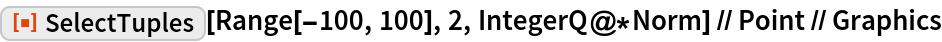Out=Properties and Relations

The main difference between Select and Tuples and SelectTuples is the amount of memory used:

 In:=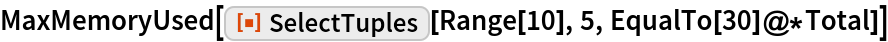Out=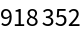Compared to naive implementation:

 In:=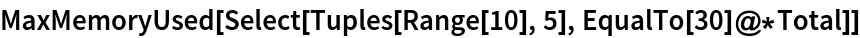Out=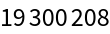Possible Issues

The length of the results might be smaller than the argument m:

 In:=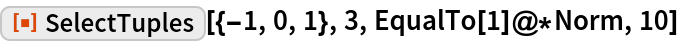Out=